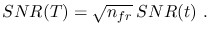# Signal-to-Noise Ratio Calculation

Under the assumption that an observation (or, equivalently, an exposure) of an object is made up of a certain number of frames (in order e.g. to reduce the total number of cosmic rays affecting the individual readouts and not to fill the potential wells of the detector' pixels, the conventions used in the following are as follows:
•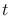: single-frame exposure time [s]
•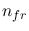: number of frames composing an oservation [pure number]
•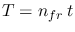: single-observation exposure time [s]
• object area : the angular size of the sky region over which a point source is spread, either due to diffraction effects or to the source being physically extended on the sky [solid angle - sr]
•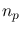: number of pixels within the object area
•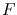: total electron counts during[ e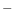]
•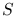: electron counts from the object during[ e]
•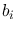: electron counts from the instrumental background per pixel during[ e]
•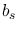: electron counts from the sky background per pixel during[ e]
•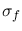: standard error of generic estimated flux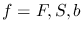[ e]
•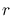: total readnoise per pixel [ erms]
•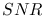: signal-to-noise ratio in the measurement of the source brightness during an observation [pure number]
Under these assumptions,can be written as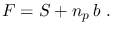(8)

The physical process of the emission of photons from an astronomical object can be statistically described in terms of a Poisson distribution. The standard error in the measurement ofis then due to the intrinsic Poisson noise associated withand to the readnoise. These two contributions sum quadratically yielding for the variance of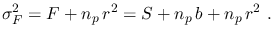(9)

Since the signalfrom the object is estimated by subtraction fromof the sky background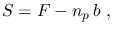(10)

the variance ofis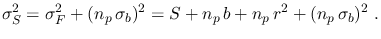(11)is then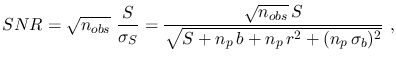(12)

while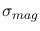is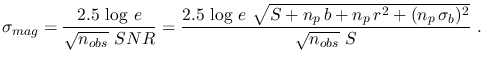(13)

The overallof a numberof repeated observations of a given field is finally given by